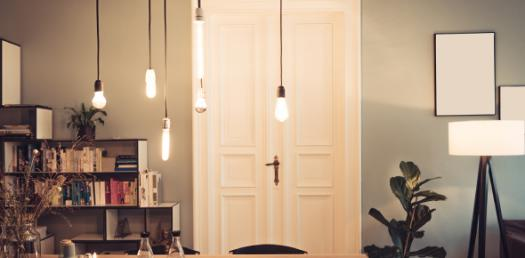# Trivia Quiz On General Lighting Basics! Test Your Knowledge

6 QuestionsSettings.

Related Topics
• 1.
A lumen is a measure of the
• A.

Strength of a light source measured at the source.

• B.

Power draw of the lamp.

• C.

Amount of usable light from a light source.

• D.

Amount of light per square foot of a work surface.

• 2.
A foot candle is a measure of the
• A.

Number of candles that would shine the same amount of light as the light source being measured.

• B.

Strength of the light measured one foot from the light source.

• C.

Amount of light shed in one square foot of area measured at a distance from the light source.

• D.

Number of feet away from a light source you are measuring.

• 3.
A foot candle is expressed in units of
• A.

Lumens per square foot.

• B.

Lumens per square meter.

• C.

Candles per square foot.

• D.

Candles per square meter.

• 4.
Which of the following is an example of “horizontal foot candles”?
• A.

The amount of light measured on the front of a computer monitor

• B.

The amount of light measured at the front of a rack aisle

• C.

The amount of light measured on a factory wall

• D.

The amount of light measured on a factory floor

• 5.
A “lux” is the metric equivalent to the US term
• A.

Lumen.

• B.

Foot candle.

• C.

Candle.

• D.

Lumen maintenance.

• 6.
Which of the above images shows a high pressure sodium lamp?
• A.

A

• B.

B